V.5 No 2 15 On reality of black holesBut the nature is know to be indifferent to any maxims expressed by this or other scientist who has an intention to establish his dictate over its laws. So, to hit the target, not the nature had to adjust itself for Einstein but Einstein had to make gross violations of its laws established and multiply checked before him by many generations of scientists. And this was revealed immediately. "From the physical point of view, the most interesting are the conservation laws in the form of trivial divergence. They always take place, if the theory was invariant relatively a continuous group of transform, i.e. that of Lee. The trouble is, for quite general gravity fields no one of these groups itself has a natural sense, as opposite to that which we have in Lorentz-invariant theories, where the group of symmetry is geometrically selected as the group of motions in space-time" [39, p. 332]. As the result, "in the particular theory of relativity, the density of energy and momentum, jointly with the strength forms the second-rank tensor field T. The divergence of this field turns to zero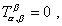(61)
 if we note all necessary kinds of energy. The full energy and full momentum can be determined with the help of this tensor by integrating over the whole three-dimensional space. Similarly, the third-rank tensor field anti-symmetrical in two indexes determines the momentum in the particular theory of relativity (RT). Only in general theory of relativity, i.e. in the classical theory of relativity (? - Authors) formulated by Einstein in 1916, there appear serious difficulties connected with the idea of energy. The roots of these difficulties are in the equivalence principles, which causes the geometrisation of gravity field. The space-time in Einstein's theory is warped and in general case whatever symmetry is lost. On the other hand, as is known, in the particular RT the energy and momentum are interrelated with the homogeneity of plane space" [39, p. 309]. Doing not going into details as to statement of the author as to special theory of relativity, we have to mark, A. Trautman rightly noticed the root of difficulty connected with the equivalency principle. Although, as we showed above, it is caused not only by warping of space-time but also by the real non-equivalency of inertial and non-inertial reference frames, similarly to, how inequinalent are in general case the reference frames accelerated and freely falling in gravity field. Just the artificially introduced analogies outwardly true for a very narrow gamut of problems, with simultaneous ungrounded extension of their applicability to the whole spectrum of reference frames, have predestined the difficulties of GR. However, even having understood it, Einstein did not try to coordinate his wishes with the reality. He undertook the way of self-willed transformation of conservation laws. "In presence of gravity field or, in other words, in the warped space-time, a simple law (61) for the density of energy-momentum tensor is not true. We have to substitute an usual derivative with that covariant, and the differential law will become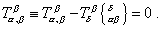(62)
 We can think the additional summand in (62) arising because in presence of gravity the energy and momentum of matter taken separately do not conserve" [39, p. 313- 314]. And again, stating so, Einstein even did not associate this with the fact that, after his own claims, the introduced regularities, in weak fields, have to be consistent with the classical theory, and not approximately but exactly. But as we now understand, Einstein inevitably had to deny the conservation laws, he was doomed to this step, as in classical physics the transition to non-inertial reference frames predetermines the conservation laws violation. But knowing this, classical physicists adhere inertial reference frames. In this transition not only conservation laws are violated but all laws of nature, in that number laws of mechanics that determine the bodies' trajectories. While Einstein, having passed to non-inertial reference frames and being compelled to violate the conservation laws, did not change the main - the laws of bodies interaction, and in this way added to his theory an appearance of some abstract non-physical geometric construction, reducing the physical values conservation to geometrical invariance of coordinate transformations. "General invariance of Einstein's theory gives, basically, not true conservation laws but four differential identities. Non the less, the full group of coordinate transformations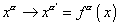contains an infinite manifold of one-parametric subgroups originated by every possible vector fields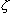(x). The gravity integral of action is invariant relatively all these one-parametric groups of coordinate transformations, and this gives an infinite manifold of weak conservation laws. Furthermore, it follows from general invariance that these conservation laws can be changed so that they to be true irrespectively of, whether the field equations are satisfied or not (power conservation laws)" [39, p. 316]. With it, "'the gravity pseudo-tensor' which Einstein introduced in 1916 … we always can turn into zero at any point, choosing the reference frame in a suitable way. So it has no sense to speak of local distribution of the energy" [39, p. 315]. But in reality this all goes basically otherwise, and we can easily show it in the above example of motion of a material point at the angle to the direction of reference frame free fall in the gravity field.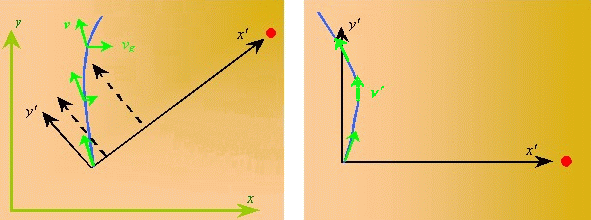a                                                                                     b
 Fig. 10. The scheme of trial body motion at an angle to the dotted reference frame freely falling in a gravity field: a - from the viewpoint of inertial (undotted) reference frame; b - from the view of non-inertial (dotted) reference frame
 In Fig. 10 we show the motion of such body whose location at the initial moment of time coincided with the origin of reference frame x'O'y' that moves with an acceleration towards the gravity centre S . We see that both in inertial and in non-inertial reference frame, at any next moment of time, not only the trajectory is warped but also the direction of attraction to the gravitating centre. Due to this, in the frame x'O'y' the body moves non-uniformly, even though at the initial moment of time we compensated the gravity field by way of choosing the reference frame. But at the next moment, to retain our calculation true, we have to account again the affection of force whose form after transition to a non-inertial reference frame will only become more complicated and will loss the properties which provided the true invariance of transformation in inertial reference frames. Here we have to mark, relativists in such cases like to speculate in the concept of locality. "If we deny the concept of inertial frame and allow in this way the arbitrary continuous transformation of coordinates, … equality of components of two vectors built at different points of space loses its invariant sense, and hence such vectors may not be compared immediately. Namely because of this reason, in general relativity we may not form new tensors from the given tensor by way of simple differentiation, and namely so we rather rarely find the invariant transformations in this theory" [33, p. 856]. We see from this citation that Einstein clearly understood how much artificial his constructions are, but again and again he reduced the problems not to real solutions but to the pseudo-physical phrasing. To understand it, it is sufficient, firstly, to draw our attention to the form of metrics used by relativists, in particular to Schwarzschild metric. It is clearly non-local and, after the author's idea, has to describe the processes at all points in the vicinity of studied gravity mass, irrespectively of the initial conditions for the studied body in the field of given mass. Thus, we see that the limitedness of invariant transformations has here no concern. Secondly, as we saw before, the field compensation cannot be fulfilled by some choice of metric. Even if we, for some specific case, introduce some curvature so that the body moved along the geodesic, having changed the initial conditions of its motion, we will have to introduce new geodesics. And if we try to consider, for example, the motion of interacting charged bodies, we will loss even the last possibility, as in non-inertial reference frames the laws of interaction will be considerably violated, and this is why classical physics prefers inertial reference frames for predicted solutions of its problems. Thus, Einstein's assurance that "this defect may be corrected through introducing the field of infinitesimal shifts", the more that "it (the field of infinitesimal shifts - Authors) replaces the inertial frame to the extent allowing to compare the vectors at the infinitely closed points" [33, p. 856], does not change the essence of problem, but substitute the study of the essence by the speculation on mathematical topics.

Contents: / 1 / 2 / 3 / 4 / 5 / 6 / 7 / 8 / 9 / 10 / 11 / 12 / 13 / 14 / 15 / 16 / 17 /